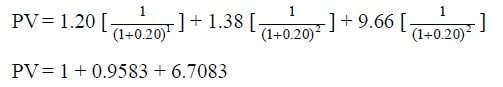Select Page

# Share Valuation Problems and Solutions

## Problem 1:

The value of ABC Company’s shares is Rs. 10 and dividend of Rs. 3 per share. It is expected that market value of share will in increase 5 percent. What is fair value of this share for one year if discount rate is 8 percent?

### Solution:## Problem 2:

The Corporation issues preferred stock that pays a dividend of Rs. 3.50 indefinitely.  Investors could invest their money at 6%.  For how much will the preferred stock sell?

## Problem 3:

The expected divided per share on the equity share of Roadking Limited is Rs. 2. The dividend per share of Road king Limited has grown over the past five years at the rate of 5 percent per year. This growth rate will continue forever in future. What is a fair estimate of the intrinsic value of the equity share of Roadking Limited if the required rate is 15 percent?

## Problem 4:

Assume that you are considering an investment in a stock that is expected to pay a constant dividend of Rs. 3 per share forever and that you will receive your first dividend payment 1 year from now. Further, you have determined that you require a 15% return on an investment in this stock. What is the value of this stock?

## Problem 5:

Dividend for first, second and third year are expected in the amount of Rs. 1, 2 and 2.50 respectively and after that dividends will grow at a constant rate of 5 % per year. Required rate is 10%. Calculate the value of stock?

##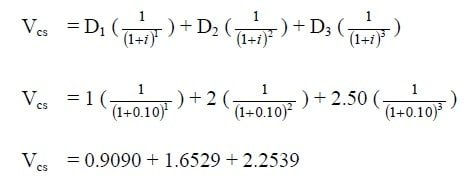Vcs    = Rs. 5.10

##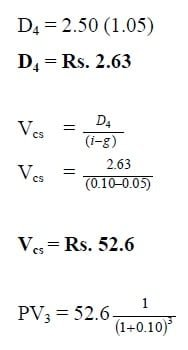PV3 = 39.52

Value of Share is 5.10 + 39.52

## Problem 6:

A preference share has a par value of Rs. 100 and a dividend rate of 10.75%. If the required rate of return is 10%, what is the share’s value?

## Problem 7:

A preference share has a par value of Rs. 100 and a dividend rate of 10.5% payable annually. If its current price is Rs. 117.52, what is its expected rate of return I f growth is 6%?

## Problem 8:

Suppose firm Z paid a dividend of Rs. 1.00 in the year that just ended. Earnings and dividends have grown several years. The required rate on the stock is 0.12 and the market price is Rs. 60 per share. Find the constant growth g?

## Problem 9:

James Company presently pays a dividend of Rs. 1.50 per share on its common shares. The company expected to increase the dividend at 12 % annual rate of the first four years and at a 13 % rate of the next two year. The face value of share of Rs. 50 is expected to growth at 15%. Assume that investor has 10 shares and sell them after 6th year, what is fair value of shares if required rate of return is 17%?

##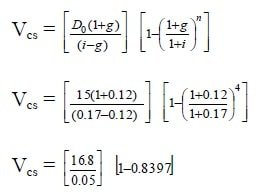Vcs    =   Rs. 53.86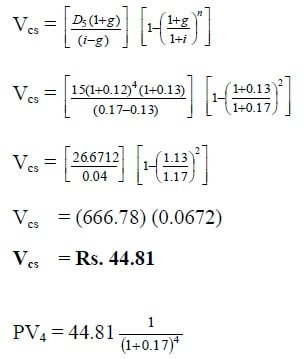PV4 = Rs. 23.91

##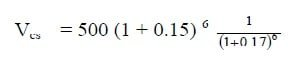Vcs    = Rs. 450.86

53.86 + 23.91 + 450.86

## Problem 10:

Suppose a firm is expected to increase dividends by 20% in one year and by 15% in two years. After that dividends will increase at a rate of 5% per year indefinitely. If the last dividend was Rs. 1.00 and the required return is 20%, what is the price of the stock?

### Solution:

D1 = 1(1.2) = Rs. 1.20

D2 = 1.20(1.15) = Rs. 1.38

D3 = 1.38(1.05) = Rs. 1.449

Find the expected future price

###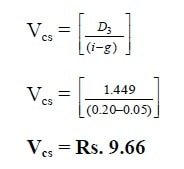Find the present value of the expected future cash flows